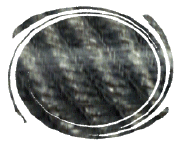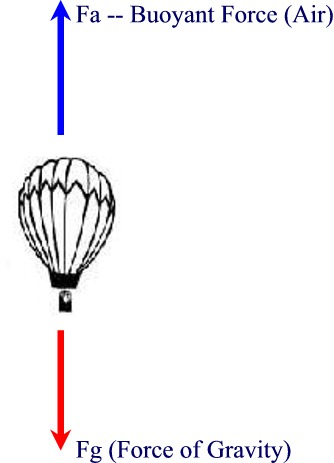#### Forces: Review Questions & Answers

1. Define Force:

Definition of Force: A push or a pull on an object.  A force is a vector which means it will have a magnitude and a direction.
Example:
The baseball was hit by the bat with a force of 320 N [East]

2. What are the units of Force in the metric system:

The metric units of Force (Also known as SI -- International System Units) are the Newtons, [N] for short.

3. Why is static friction larger than kinetic friction

Static Friction is larger than kinetic (also called moving) friction.  This is due to the fact that once the object is set in motion it takes less inertia to keep it in motion. (Newton's First Law)

4. What are three factors affecting the Force of friction on a surface?

The three factors that affect the force of Friction (Ff) are:

• The roughness of the surface (coefficient of friction -- µ)
• mass of the object (therefore the Force of gravity or weight, because F= m X g)
• Presence of a lubricant
•

5. What is a lubricant and how does it work?

A lubricant is a substance that, when applied to a surface, it will decrease the Force of friction acting on that surface.

How a lubricant works:

Let's look at two different surfaces viewed under a microscope

 Type of Surface Example Microscopic Description Picture Action of Lubricant Rough Surface Rubber Lots of "bumps", crevices, grooves and imperfections -Fills gaps, bumps and imperfections -- good, thick lubricant needed Smooth Surface Glass few imperfections-- thin lubricant is sufficient

6. In late august, many times we can see showers of "falling stars" when looking at the night sky.  Explain this phenomenon with the knowledge you gained about Friction and Newton's Laws.

When a metereorite enters a planet's atmosphere it becomes a shooting star because of the friction forces it encounters as it rubs against layers and  layers of air. A lot of heat is generated which (luckily for us) in turn ignites the metereorite.

7. Mary Poppins uses an umbrella to "fly" from place to place and gently land on roof tops and so on.
Explain if this is really possible using your Physics Knowkedge.

Mary Poppins uses her umbrella as a parachute.

When a parachute is opened it will slow down  the fall by increasing the air resistance and thus attenuating (minimizing)  the force of gravity that is pulling down on it.

The forces on the parachute (umbrella) are not balanced because it continues to accelerate down.8. Summary of Law of Inertia and Newton's Second Law

 Situation Forces Acting on Object Resulting Motion of ObjectObject is at rest balanced forces stays at rest Fnet = 0   and   a = 0 Object is in motion balanced forces keeps moving at constant speed Fnet ≠ 0    and   a ≠ 0 Object is at rest Unbalanced forces accelerates in the direction of unbalanced force

9. "Wear Seat Belts"!!

This is good advice because not only you will avoid getting a ticket and losing demerit points if you don't but also because it will prevent you from hitting your head against the windshield if the brakes are suddenly applied.

According to the Law of Inertia (Newton's Third Law), objects in motion will tend to preserve their state of motion.

Therefore, if the brakes were suddenly applied and you didn't have a seat belt, you would keep moving with the same speed of the car before the brakes were hit.  The only thing that would stop you then would be the windshield.

10. If a 2.5 kg bag of potatoes were pushed on a (frictionless) table with a force of 5.0 N, What would be the resulting acceleration?

Given: m = 2.5 Kg

Find: a

Solution:  F = m X a
F = 5.0 N

:.  a = F/m

a = 5.0 N / 2.5 Kg

a = 2.0 m/s2